Grammar: Application
 Over the past thirty years, two separate fundamental approaches to graph application have been derived. The two approaches have been distinguished from one another as: algebraic versus algorithmic, gluing versus connecting, or context-sensitive versus context-free and both have been incorporated into GraphSynth. Figure 1 shows an overall flowchart for this application procedure. This flowchart captures in a rigorous way all graph transformations that may occur; addition, subtraction, or modification to nodes, arcs, and labels. The thick dotted line in the figure shows the algorithmic path of the application procedure while the long ‘U’ shaped lines indicate subgraph distinctions, where A Ì B indicates that A is a subgraph of B. The input to application includes the three items on the left of Figure 1. The rule and the L-mapping (or locations) are the result of the previous choose function that results from recognition (see Generation for description). These along with the host graph, G, represent the first elements of what is commonly referred to as the Double-Pushout method, which is discussed in detail in the following subsection. Following the pushout that removes elements from the host (Step 1 in Figure 1); a second pushout adds new elements to the graph (Step 2 in Figure 1). This concludes the traditional algebraic approach to graph transformation; however, for completeness, a third step is implemented to accomplish Free-Arc Embedding of possible dangling arcs. This third step (Step 3 in Figure 1), based on a previous approach referred to as edge-directed Neighborhood Controlled Embedding, is improved upon in the implementation of GraphSynth. It is described in more detail below.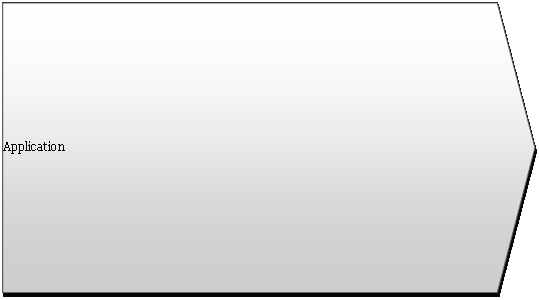Figure 1: The  application of a graph grammar rule takes as input: a rule to apply, how that rule’s L is mapped into the host, and the host graph.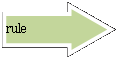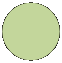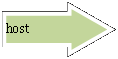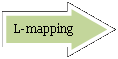LK
 R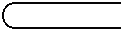H
 G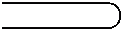D
 H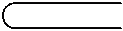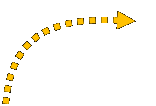Embedding rules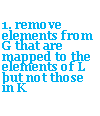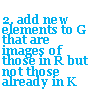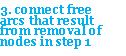The representation of embedding rules is accomplished by simply saving the these variables within a grammar rule. Any of the first four elements of this rule representation may be left blank to specify that the rule is not concerned about a particular quality. This embedding method differs from previous by reconnecting the free arcs as opposed to creating new arcs. An addition of a seventh quality, allowDuplication gives the approach the ability to create more arcs than what is originally contained in the host. Additionally, if none of the first four qualities of the rule are specified, then the rule will be matched to all free arcs (this is seen in the example of Figure 2). Any free arcs that meet no conditional rules are deleted as a final step in creating the new host, H'. It is important to note that the order of the rules is important. Any combination of embedding rules can be used to identify a free arc. If two rules are recognized with the same free arc only the first one will modify it, as the arc will no longer be ‘free’ after the rule is applied; however, in cases when allowDuplication is set to true, a copy of the free arc will remain for the other rules to operate on. As a result, when a rule duplicates an arc in this way, it does not prevent further rules from being recognizing the free arc.

## The Algorithmic Free-Arc Embedding

The second approach to graph application is often referred to as the ‘algorithmic approach’ or the ‘connecting approach.’ In many instances the two approaches are able to perform identical graph modifications, and in general the DPO method is preferred for its ease of representing graph modifications by simple graph subtractions and additions. The algorithmic method is ‘context-free’ which on a low-level relates to the lack of the common or context graph, K, but on a higher-level this means that the rules can be applied in more generic way. Consider the example in Figure 2. The three nodes of the left hand side, L, are removed leaving two dangling arcs and are replaced by two new nodes and a single connecting arc. The two dangling arcs are then connected following a condition that informs the transformation process of where these arcs are connected.

 The approach used in GraphSynth is referred to as Free Arc Embedding and takes advantage of our ability to handle dangling arcs. Following the algebraic approach (see Figure 1), we gather the dangling arcs that were connected to the nodes deleted in the first part of DPO and check them with specific embedding conditions of the form: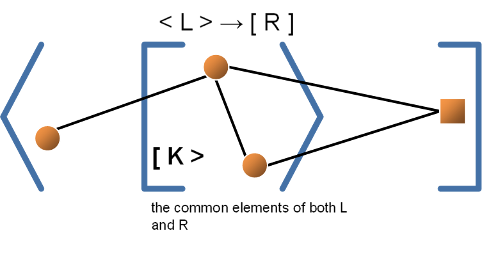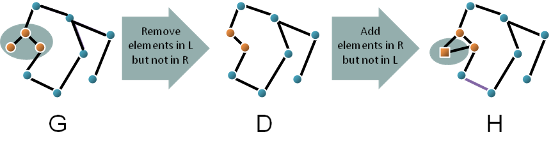Figure 2: An example of a DPO rule application. First elements are deleted followed by the addition of new elements.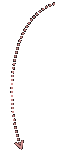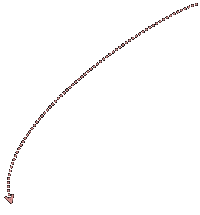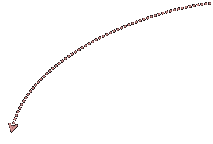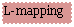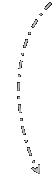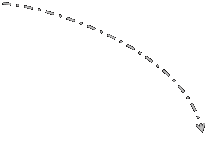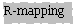## The Algebraic Double Pushout Method

In general, the description of grammar rules contains some overlap in the two graphs L and R. This overlap is referred to as K, and indicates what parts of the L-mapping are to be kept through the rule application. Any elements (nodes, arcs, or labels therein) that are to be deleted in this rule transformation are therefore stored in L but not in K. One could view the DPO method as a function:
H=G – (L – R) + (R – L)
where the parts of L that are not in R are first deleted (L-R), followed by the addition of elements in R but not in L (R-L). This method of adding elements via graph transformation is sometimes referred to as the ‘gluing’ method or the context-sensitive method given this approach at adding new elements to a host. One final change that can be made in this part of application is to modify the labels in the K elements. This modification happens in a similar way to the overall rule operation but is applied on a set as opposed to a graph. Within each element in K, we identify the labels that exist when in L that are no longer stated when in R and delete them. This is then followed by adding the new labels that are in R but not in L; the common labels are left alone. In terms of implementation, we represent L and R as independent graphs. As such there is no true intersection graph K that results. What is done to represent the K elements is to require that arcs and nodes with identical ‘names’ in L and R are the same element, when the user constructs a rule. This name tag is separate from labels which are used to describe qualities in the graph. Name is an implementation fix to storing K elements in two separate instances. As a result, the aforementioned modification of K labels can be easily performed. An example DPO rule application is shown below in Figure 2.Figure 3: An example of free arc embedding. The embedding rule specifies only an RNodeName, causing all free arcs to be connected to node III.if free arc    ((contains label, freeArcLabel)                        AND                        (was previously connected to the deleted node matched                                   with the L node, LNodeName)                        AND                        (is currently attached to a neighbor node with label,                                   neighborNodeLabel))                        AND                        (is in the direction of originalDirection)) then               (connect to node, RNodeName in the direction of newDirection).